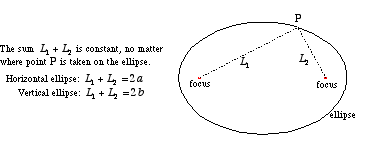# drawing reflected rays in ellipse(optical property

hi there,i have some problems coming up with an idea how to demonstrate optical properties of an ellipse in openGL.I managed to draw an ellipse to represent the case when there’s only one reflection.As you know,a ray is coming out of one focus and has to go through the other one.Any ideas how it could be done for lets say n reflections aka more than two rays.it should look something like this:
After one reflection :After more reflections:AND THIS IS MY CODE FOR DRAWING THE ELLIPSE AND THE CASE WHEN THERES ONE REFLECTION(2 rays):
glBegin(GL_POINTS);/drawing an ellipse/

while(i<360)
{

``````  float degInRad = i*RADIAN;
``````

i+=0.1;
}

glEnd();

c=sqrt(aa-bb);/foci/

glColor3f(0.5,0.5,0.0);

``````float f=angle*RADIAN;
``````

int sign=1;

if(angle>180 && angle<=360) sign=sign*(-1);/drawing on negative y-axes/

float xe=cos(f)a;
float ye=sign
sin(f)*b;

glBegin(GL_LINES);
glVertex3f(-c,0.0,0.0);
glVertex3f(xe,yesign,0.0);
glVertex3f(xe,ye
sign,0.0);
glVertex3f(c,0.0,0.0);
glEnd();

-And this is how the image looks like when i run it with my program where a=10,b=4 and angle=30 degrees